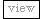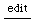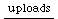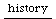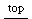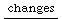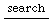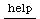## Switch

This class allows SC to emulate a "switch" statement which you find in other languages (C, Java, JavaScript, ...):

Copy the section between the "—-" and save it as Switch.sc. Put it into your library.
```  ------ Switch.sc ----------

/*
Emulates a switch statement
*/

Switch {
var <onValue, hasExecuted;

// Instance Creation
*on {arg aValue;
^this.new.initialize(aValue);
}

// initialize-release
initialize {arg aValue;
onValue = aValue;
hasExecuted = false;
}

// case stuff
case {arg test, block;
hasExecuted.if({^this});
test.value(onValue).if({this.execute(block)});
^this
}

execute {arg block;
block.value(onValue);
hasExecuted = true;
}

fallThrough {
hasExecuted = false;
}
}
----- end Switch.sc --------
```

Here's an example of how to use it:

var a, b;
a = MouseX.new(-2, 3);
b = MouseY.new(1, 8);

Switch.on(b.poll).
case({(a.isNil)}, {nil}).
case({(a.poll > 2)}, {arg g; g.poll.squared;}).
case({(a.poll == 0)}, {arg g; g.poll.sqrt;}).
case({(a.poll -1)}, {arg g; g.poll.post;});